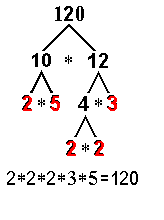Our Address: FZ LLC G 37 & G 34, Block 13, Al Sufouh Road Knowledge Park, Dubai PO Box : 501703

# Number Properties – FactorsAs promised in the last post, here we are going to go deeper into number properties tested very frequently on the GMAT. We first begin with prime numbers.

Prime Numbers

A number is prime if it has only two factors: ‘1’ and the number itself, e.g. ‘2’. A number which has more than two factors is called composite, e.g. ‘4’. The concept of prime numbers is applicable only to positive integers. ‘1’ is itself neither prime nor composite. The first few prime numbers are 2, 3, 5, 7, 11, 13, 17, 19, 23, 29 and 31… Once you know these basic points about prime numbers, the next important concept is prime factorization.

Prime Factorization

Every positive integer can be written in a unique way as a product of prime numbers; this is called its prime factorization.

How to do prime factorization

Let’s say we want to find the prime factors of 120. We start testing all integers to see if and how often they divide 120 and the subsequent resulting quotients evenly.

120 ÷ 2 = 60; save 2

60 ÷ 2 = 30; save 2

30 ÷ 2 = 15, save 2

15 ÷ 2 = 7.5, not evenly so try next highest number, 3

15 ÷ 3 = 5, save 3,

5 ÷ 5 = 1; save 5

Therefore 120 = 23 x 3 x 5

Using a “factor tree”, your work would look like thisPrime factorization helps us deduce a lot of things about the number. Let’s look at one of the most important deductions.

Number of factors

Once we have the prime factorization of a number, we can find the total number of factors of a number very quickly (this would otherwise be quite tedious, since we want ‘allthe factors).

Let’s say you are asked to find the total number of factors of 120.

First of all, the prime factorization of 120 = 23 x 3 x 5

To find the total number of factors

– Find the exponents of each prime number in the prime factorization ( here 3, 1 and 1)

– Add one to each value (so 4, 2, 2)

– Multiply the numbers

So the number of all factors of 120 = 4x2x2 = 16

Another variant of this is to find the number of odd and even factors. For odd factors, we leave out the exponent of 2, since even a single ‘2’ on multiplication would give an even number. So, the number of odd factors = 2×2= 4. And for finding the number of even factors, we simply subtract the number of odd factors from the total number of factors (here 16 – 4 = 12).

At Option Training Institute, it is our constant endeavor to help people do well in Quant even if they found Math scary in grade school.